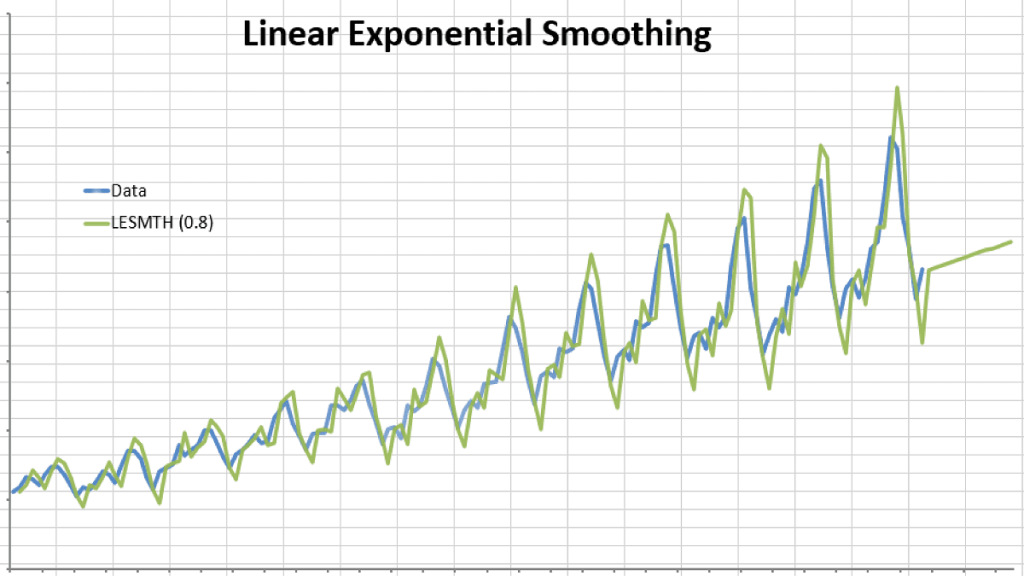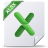# Brown’s Linear Exponential Smoothing

This is another method of double exponential smoothing function, but it has one smoothing factor:

$S_1^{‘}=X_1$

$S_1^{”}=X_1$

$S_{t>1}^{‘}=\alpha X_t + (1-\alpha)S_{t-1}^{‘}$

$S_{t>1}^{”}=\alpha S_{t}^{‘}+(1-\alpha)S_{t-1}^{”}$

$a_{1<t<T}=2 S_{t}^{‘}-S_t^{”}$

$b_{1<t<T}=\frac{\alpha}{1-\alpha}\times (S_t^{‘}-S_t^{”})$

$Y_{T+m}=a_T+m\times b_T$

Where:

• $\alpha$ is the smoothing factor ($0 \prec \alpha \prec 1$)

Brown’s double exponential smoothing takes one parameter less than Holt-Winter’s function, but it may not offer as good a fit as that function.

Example 1 :

Let’s use the same example in Holt-Winter’s double exponential and compare the optimal sum of the squared error.

How do we find the best smoothing factor ($\alpha$)?

We use the same method to select the alpha value that minimizes the sum of the squared error. For the example sample data, the alpha is found to be 0.8

## Files Examples